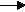gcsescience.com                                       6                                       gcsescience.com

What are Ionic Equations?

The displacement reactions can be written as ionic equations.
In the example using iron and copper(II) sulfate (previous page)

iron copper(II) sulfateiron sulfate  copper.
Fe(s)    +     CuSO4(aq)FeSO4(aq)  +     Cu(s)

Copper(II) sulfate and iron sulfate are ionic compounds.
When they are dissolved in water
the ions become separated by the water molecules.

If we write the equation showing the ions we have

Fe(s)  +  Cu2+(aq)SO42-(aq)Fe2+(aq)  +  SO42-(aq)  +  Cu(s)

In going from reactants to products
iron metal - Fe(s)  has become iron ions - Fe2+(aq)
copper ions - Cu2+(aq)  have become copper metalCu(s)
sulfate ions - SO42-(aq) are not changed during the reaction.
S
ulfate ions are the same on the left and the right
side of the arrow. Ions which do not change
during the reaction are called spectator ions.

Spectator ions can be left out of the equation, giving

Fe(s)  +  Cu2+(aq)Fe2+(aq)  +  Cu(s)

This is the ionic equation
for the reaction between iron and copper(II) sulfate.
Iron is oxidised and copper is reduced.

Similarly, the reaction
between tin and lead chloride may be written as

Sn(s)   +   Pb2+(aq)Sn2+(aq)   +   Pb(s)

Tin is oxidised and lead is reduced.

gcsescience.com    The Periodic Table    Index    Reactivity Series Quiz    gcsescience.com